ML Aggarwal Class 6 Solutions for ICSE Maths Model Question Paper 3 acts as the best resource during your learning and helps you score well in your exams.

## ML Aggarwal Class 6 Solutions for ICSE Maths Model Question Paper 3

Section-A
Questions 1 to 8 is of 1 mark each.
Choose the correct answer from the given four options (1 to 8):
Question 1.
The difference between the place value and face value of 5 in the numeral 70542 is:
(a) 0
(b) 42
(c) 495
(d) 537
Solution: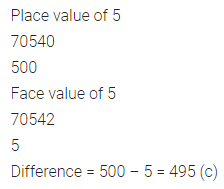Question 2.
he sum of the successor of 99 and the predecessor of 101 is
(a) 198
(b) 200
(c) 201
(d) 199
Solution:Question 3.
If x and y are two co-prime numbers, then their LCM is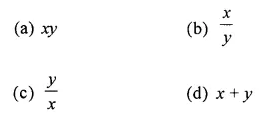Solution:Question 4.
Which of the following statement is true?
(a) |15 – 6| = |15| + |-6|
(b) Additive inverse of-3 is 3
(c) -1 lies on the right of 0 on the number line
(d) -4 is greater than -3
Solution:Question 5.
The fraction equivalent to $$\frac{360}{540}$$ isSolution:Question 6.
Which of the following numbers is divisible by 6?
(a) 5372
(b) 6495
(c) 7632
(d) 7568
Solution: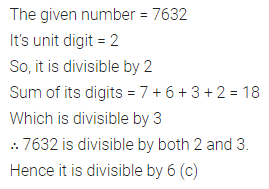Question 7.
5 kg 5g is equal to
(a) 5.5 kg
(b) 5.05 kg
(c) 5.005 kg
(d) 5.0005 kg
Solution:Question 8.
The ratio of a number of girls to the number of boys in a class is 7 : 5. If there are 15 boys in the class, then the number of girls in the class is
(a) 14
(b) 21
(c) 28
(d) 36
Solution: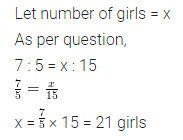Section-B
Questions 9 to 14 are of 2 marks each.
Question 9.
Write the greatest and the smallest 4-digit numbers using four different digits with the condition that the digit 4 occurs at tens place.
Solution:Question 10.
Find the prime factorisation of 980.
Solution: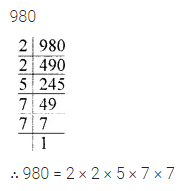Question 11.
Find the value of-15 – (-2) – 71 – (-8) + 6.
Solution: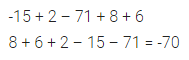Question 12.
What fraction of the given figure is the shaded part?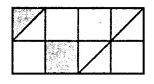Solution:Question 13.
Write the mixed fraction $$7 \frac{3}{40}$$ as a decimal number.
Solution:Question 14.
The length of a pencil is 14 cm and its diameter is 7 mm. What is the ratio of the diameter of the pencil to that of its length?
Solution: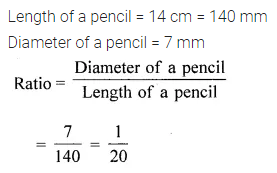Section-C
Questions 15 to 24 are of 4 marks each.
Question 15.
Estimate the product 2459 x 653 by rounding off each factor to its
(i) greatest place
(ii) nearest hundreds.
Solution: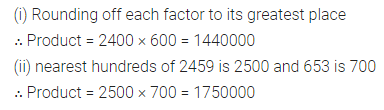Question 16.
By using distributive laws, find 257 × 1007.
Solution: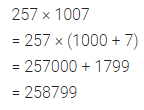Question 17.
Using number line, subtract (-3) from (-8).
Solution: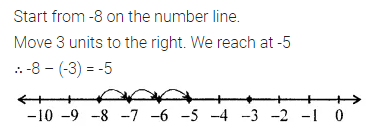Question 18.
Find the greatest number that will divide 76, 113 and 186 leaving remainders 4, 5 and 6 respectively.
Solution: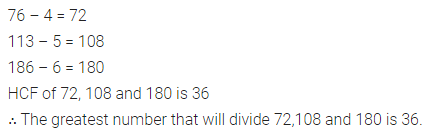Question 19.
Arrange the following integers in descending order:
-506, 2376, 2367, -311, -509, 245.
Solution: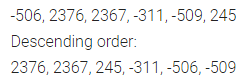Question 20.
Arrange the following fractions in ascending order $$\frac{5}{12}, \frac{1}{4}, \frac{7}{8}, \frac{5}{6}$$.
Solution: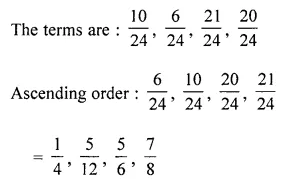Question 21.
Simplify: $$3 \frac{5}{6}+4 \frac{3}{4}-5-1 \frac{3}{8}$$.
Solution: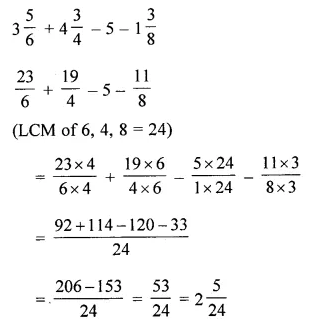Question 22.
Write all possible natural numbers using the digits 3, 0, 8. Repetition of digits is not allowed. Also, find their sum.
Solution: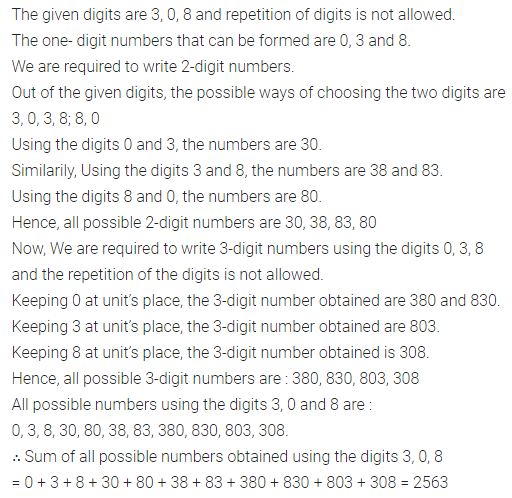Question 23.
Munna and Munni are aged 14 years and 10 years. Their mother wants to divide ?180 between them in the ratio of their ages. How much does each get?
Solution: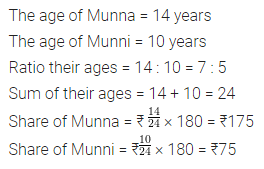Question 24.
Do the ratios 15 cm to 3 m and 15 seconds to 3 minutes form a proportion? Justify your answer.
Solution: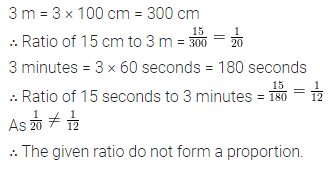Section-D
Questions 25 to 29 are of 6 marks each.
Question 25.
To stitch a shirt, 2 m 25 cm cloth is needed. Out of 30 m cloth, how many shirts can be stitched and how much cloth will remain?
Solution:Question 26.
Four bells are ringing at intervals of 40, 30, 36 and 45 minutes respectively. At what time will they ring together again if they start ringing simultaneously at 9 A.M.?
Solution:Question 27.
I bought fruits worth ₹$$27 \frac{3}{4}$$ and vegetables worth ₹$$10 \frac{1}{2}$$. If I gave a fifty-rupee note to the shopkeeper, how much will I get back?
Solution: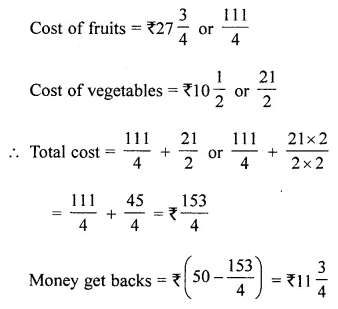Question 28.
Javed purchased vegetables weighing 10 kg. Out of this, 3 kg 450 g was potatoes, 2 kg 10 g was onions, 1 kg 750 g was tomatoes and the rest were green vegetables. What was the weight of green vegetables?
Solution: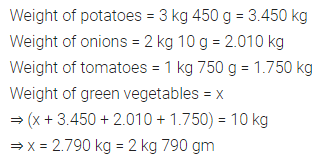Question 29.
To get a closer finish in a 100 m race, the runners are given different starting positions, depending upon how fast they can run. A starting position of +10 means that the runner starts 10 m in front of the starting line and -5 means that the runner starts 5 m behind the start line. The competitors starting positions are :
Abbas 0 Mohan -3 Rishi -20
Pete  + 10 Tarush + 15 Sahel + 5
(i) Who starts farthest from the finish line?
(ii) How far does Tarush have to run to reach the finish line?
(iii) How far Mohan has to run to reach the finish line?
(iv) How many metres are there between the starting positions of Rishi and Peter?
(v) What value is added if the students are asked to run 1 km daily?
Solution: